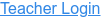PhET Interactive Simulations

• Solutions
• Molarity
• Moles
• Volume
• Solubility
• Saturation

### Description

What determines the concentration of a solution? Learn about the relationships between moles, liters, and molarity by adjusting the amount of solute and solution volume. Change solutes to compare different chemical compounds in water.

### Sample Learning Goals

• Describe the relationships between volume and amount of solute to concentration.
• Explain how solution color and concentration are related.
• Calculate the concentration of solutions in units of molarity (mol/L).
• Use molarity to calculate the dilution of solutions.
• Compare solubility limits between solutes.
Version 1.3.2